How to Concatenate Cells and Add Different Separators in Excel

Sometimes we want to concatenate words which are separated into different cells into one big column, how can we do? Now we will share you some small tips to concatenate words in Excel, and you can also add different separators between words if you like.

Concatenate cells by CONCATENATE Function

This function is very useful for concatenating words. Here are some samples to demonstrate this function.

a. Insert space between words by CONCATENATE FUNCTION.

Space is a quite common separator for concatenate words. See below table, we have two columns, and Hello word is listed in column A->A1, world in column B->B1; Good in column A->A2, Evening in column B->B2.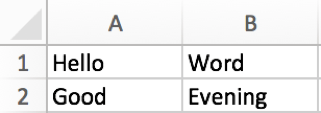Step 1: In cell C1, enter the formula =CONCATENATE(A1,” “,B1).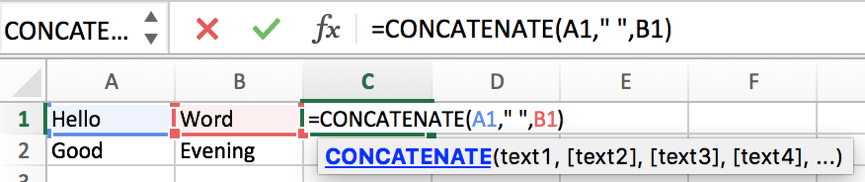Step 2: Press Enter to get the result.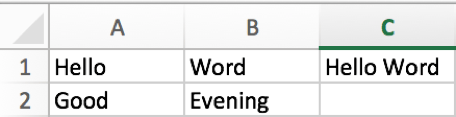Step 3: Copy cell C1 to C2. Words are merged into one cell properly.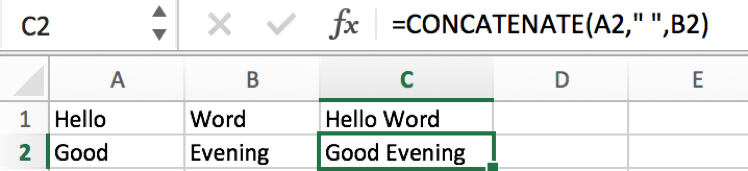b. Insert point “.” between words by CONCATENATE FUNCTION.

A point can be used for concatenate the first name and the last name which are listed in different cells.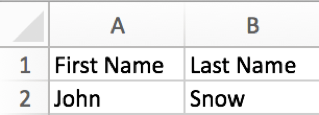We can repeat above steps to concatenate the first name and the last name, but as in this case, we want concatenate names by “.”, so we need to update the formula.

Enter =CONCATENATE(A2,”.”,B2). You can find that we replace “ ” with “.” in the formula.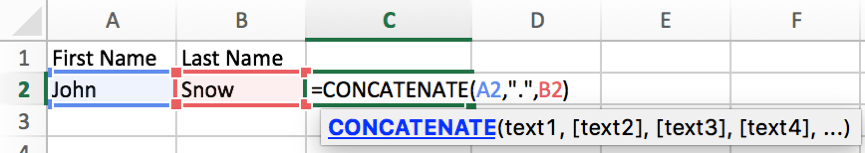Now full name is displayed in C2 properly.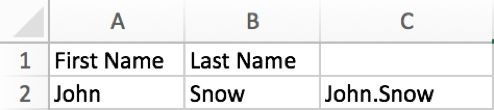c. Insert dash “-” between words by CONCATENATE FUNCTION.

This separator is often used to connect date as a period. See below example.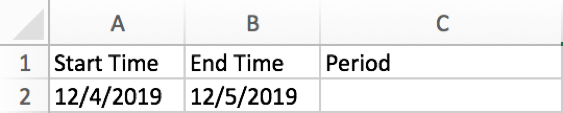Enter =CONCATENATE(A2,”-“,B2). Now you may know that we can enter any separator we want into the quotation marks to connect words.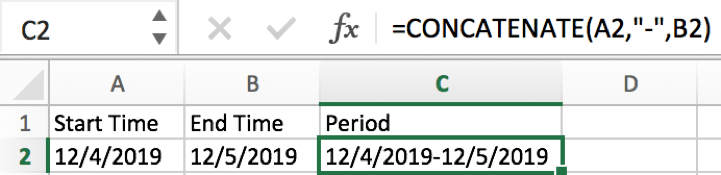Notes: we have to change the format to Text for date, otherwise the concatenate period may be displayed improperly.

Concatenate words by & symbol

You can also use a & symbol to do connection if you forget to use CONCATENATE function in Excel. Here is a simple way to connect words.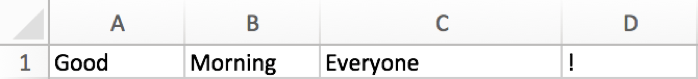In E1, enter =A1&” “&B1&” “&C1&” “&D1. There is a space between double quotes. That’s because we want to use a space to separate each word.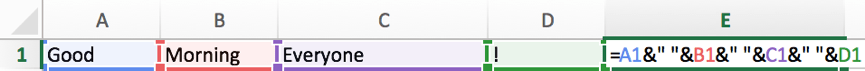A complete sentence is displayed in E1.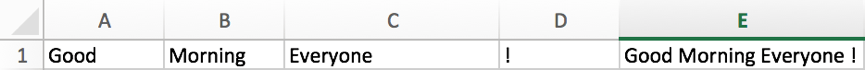Related Posts

Filter Data by Column and Sort by Row

This post will guide you how to use the FILTER function to filter a value by column and then sort data by row in Microsoft Excel. You can use the following formula based on the SORT function in combination with ...

Filter And Transpose Data From Horizontal To Vertical

This post will show you how to use Filter function and in combination with Transpose function to filter data from horizontal and transpose data as vertical in Microsoft Excel. You can refer to the below general formula based on TRANSPOSE ...

VLOOKUP Formula | Faster Trick with 2 VLOOKUPS

This post will guide you how to use 2 VLOOKUPS function to looking up data entries from a given range of cells in Microsoft Excel. VLOOKUP with 2 lookups can be faster than a single VLOOKUP in certain scenarios. The ...

Extract Unique Items From A List

This post will guide you how to extract unique itmes from a given list in Microsoft Excel. How to create a newly formula to get unique values from a range cells in Excel. The unique list of items is the ...

Extract substring In Excel

This post will guide you how to use Excel's MID function is a quick and easy way to extract pieces from your text. Use the Excel formula to extract a substring with MID. Note: If you want to extract just ...

How to Use 3D SUM Multiple Worksheets

To sum a range of numbers is straightforward for most Excel users, but do you know how to establish a 3D reference to total the same range of numerous sheets. In this post, I will present the steps for this ...

Extract all the matches with helper Column

With Excel's powerful functions IF, INDEX, and MATCH, we can find exactly what you're looking for with a few clicks of the mouse. This step-by-step tutorial will show how easy it is to extract data using these tools and more! ...

If Cell is This Value or That Value

IF function is frequently used in Excel worksheet to return you expect “true value” or “false value” based on the result of logical test. If you want to see if a cell is A or B, and if one of ...

If Value is Greater Than A Certain Value

IF function is frequently used in Excel worksheet to return you expect “true value” or “false value” based on the logical test result. If you want to see if a value in one cell is greater than a specific value, ...

If Cell is Not Blank

IF function is frequently used in Excel worksheet to return you expect “true value” or “false value” based on the result of created logical test. If you want to see if a cell is blank or not, and leave some ...

Sidebar